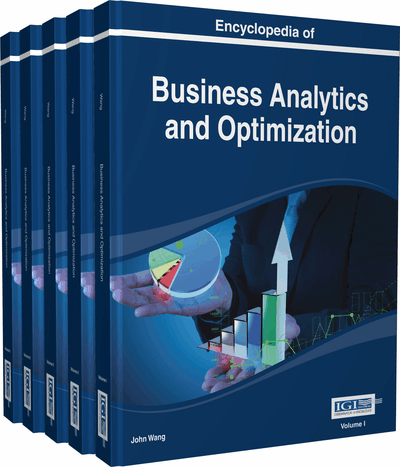# Nonlinear Efficiency in DEA Relative to “Ideal Reference”

P. Sunil Dharmapala (College of Economics & Political Science, Sultan Qaboos University, Sultanate of Oman)
DOI: 10.4018/978-1-4666-5202-6.ch146

## Abstract

Several researchers in the past have emphasized the importance of computing efficiency measures in Data Envelopment Analysis (DEA) relative to a best-practice benchmark. Thompson et al. (1995) introduced a nonlinear efficiency measure with linked-cone (LC) assurance-regions (AR) in DEA. In this paper, we compute Thompson-Thrall's measure vis-à-vis linear efficiency measures of CCR (Charnes et al., 1978), BCC (Banker et al., 1984), CCR/AR and BCC/AR (Thompson et al., 1992), relative to “ideal reference”- an industry average. We demonstrate the computations in an application to a set of banks and show that the nonlinear measure is stricter than the linear measures.
Chapter Preview
Top

## Introduction

Since the original publication on Data Envelopment Analysis (DEA) by Charnes et al. (1978) measuring the efficiency of decision making units, there has been a rapid and continuous growth in this area of study. A considerable amount of research publications have appeared, a significant portion of which focusing on DEA applications of efficiency and productivity in the banking sector. For example, a comprehensive survey of literature on bank efficiency could be found in Fethi and Pasiouras (2010). They have examined bank branch efficiencies in more than 30 studies over the period 1998-2009. All these studies are using DEA to estimate bank efficiency. Thompson et al. (1995) introduced nonlinear LC-AR efficiency measure to DEA literature, where they discussed the single-output multiple-inputs model subject to linked-cone (LC) assurance-regions (AR).

A major criticism leveled against DEA is that DEA measures only relative efficiency of decision making units (DMUs) participating in the study. While highlighting this as a drawback in DEA methodology, some authors in the past have suggested incorporating best-practice efficiency analysis by including an industrial benchmark among the DMUs. Lei Li et al. (2013), Dharmapala et al. (2007), Cook and Zhu (2006), Zhu (1996), Thanassoulis and Dyson (1992), and Golany (1998) are among those suggested. In this paper, we compute the nonlinear LC-AR measure against the linear radial measures of CCR (Charnes et al., 1978), BCC (Banker et al., 1984), CCR/AR and BCC/AR (Thompson et al., 1992), relative to an “ideal reference”- an industrial benchmark. We demonstrate the computations in an application to a set of banks and show that the nonlinear measure is stricter. To our knowledge, we may be the first to carry out such a comparative study.

## Key Terms in this Chapter

Linked Cone Assurance Regions: Restrictions on input-multipliers and output-multipliers in the form of constraints (2) and (3) that lead to the construction of input-cone and output-cone in CCR/AR and BCC/AR models along with linked-cone (4) in TT/LC-AR model ( Thompson et al., 1995 ).

Efficient DMU: A DMU with maximum Z-score 1, provided that all input and output slacks in the optimal solution (in TT/LC-AR, CCR/AR or BCC/AR model) are zero. (If max Z < 1, the DMU is inefficient).

Constant Returns: Neither (i) nor (ii) in “Variable Returns” described below.

Input-Oriented Model: Find the best set of weights to maximize the weighted ratio of outputs-to-inputs at fixed levels of inputs, subject to the constraint that no ratio is more than unity.

Nonlinear LC-AR Efficiency Measure: Efficiency score computed with the nonlinear objective function subject to “Linked Cone Assurance Regions.”

Ideal Reference: An additional DMU that is included among other DMUs, and it represents the ‘Industrial Average’ which is treated as the best-practice benchmark. This DMU may be a hypothetical entity.

Variable Returns: For a fixed DMU c with input-output vector (X c , Y c ), (i) it is possible to achieve a more than proportionate increment in Y c when X c is augmented (Increasing returns), or (ii) it is possible to achieve a less than proportionate decrement in Y c when X c is decremented (Decreasing returns).

Multipliers: Weights v i on inputs and u k on outputs that need to be determined by solving the respective programming model TT/LC-AR, CCR/AR or BCC/AR.

Price/Cost Data: The range of values of multipliers defined by the ratio of Revenue-to-Cost based on market prices.

## Complete Chapter List

Search this Book:
Reset